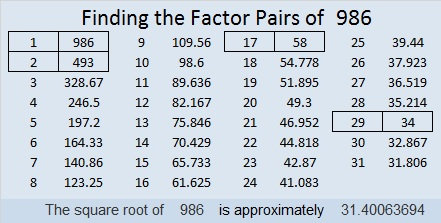# 986 and Level 1

Today’s puzzle looks like a simple holiday napkin, and it’s actually quite simple to solve. Even kids who have learned how to multiply but haven’t even heard of division could solve this puzzle.Print the puzzles or type the solution in this excel file: 10-factors-986-992

986 looks the same upside-down as it does right-side up, so it is a strobogrammatic number.

31² + 5² = 986
25² + 19² = 986

986 is the hypotenuse of FOUR Pythagorean triples:
264-950-986
310-936-986
680-714-986
464-870-986

986 looks interesting in some other bases:
12421 BASE 5
TT in BASE 33 (T is 29 base 10)
T0 in BASE 34

• 986 is a composite number.
• Prime factorization: 986 = 2 × 17 × 29
• The exponents in the prime factorization are 1, 1, and 1. Adding one to each and multiplying we get (1 + 1)(1 + 1)(1 + 1) = 2 × 2 × 2 = 8. Therefore 986 has exactly 8 factors.
• Factors of 986: 1, 2, 17, 29, 34, 58, 493, 986
• Factor pairs: 986 = 1 × 986, 2 × 493, 17 × 58, or 29 × 34
• 986 has no square factors that allow its square root to be simplified. √986 ≈ 31.4006